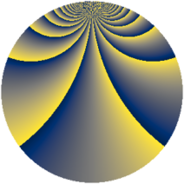# Properties

 Label 3630.2.biLevel $3630$ Weight $2$ Character orbit 3630.bi Rep. character $\chi_{3630}(23,\cdot)$ Character field $\Q(\zeta_{44})$ Dimension $5280$ Sturm bound $1584$

# Related objects

## Defining parameters

 Level: $$N$$ $$=$$ $$3630 = 2 \cdot 3 \cdot 5 \cdot 11^{2}$$ Weight: $$k$$ $$=$$ $$2$$ Character orbit: $$[\chi]$$ $$=$$ 3630.bi (of order $$44$$ and degree $$20$$) Character conductor: $$\operatorname{cond}(\chi)$$ $$=$$ $$1815$$ Character field: $$\Q(\zeta_{44})$$ Sturm bound: $$1584$$

## Dimensions

The following table gives the dimensions of various subspaces of $$M_{2}(3630, [\chi])$$.

Total New Old
Modular forms 16000 5280 10720
Cusp forms 15680 5280 10400
Eisenstein series 320 0 320

## Trace form

 $$5280q + 8q^{7} + O(q^{10})$$ $$5280q + 8q^{7} + 36q^{10} + 72q^{13} + 528q^{16} - 16q^{21} - 84q^{22} - 16q^{25} + 8q^{28} - 16q^{31} + 8q^{33} + 16q^{36} - 16q^{37} - 32q^{42} - 48q^{43} + 32q^{45} + 16q^{46} - 128q^{51} + 16q^{52} + 100q^{55} + 32q^{57} + 56q^{58} + 32q^{61} + 180q^{63} - 8q^{66} - 48q^{67} - 64q^{70} + 56q^{73} - 64q^{75} - 16q^{78} - 16q^{81} - 16q^{85} + 64q^{87} + 4q^{88} - 32q^{90} - 528q^{91} + 88q^{93} + 40q^{97} + O(q^{100})$$

## Decomposition of $$S_{2}^{\mathrm{new}}(3630, [\chi])$$ into newform subspaces

The newforms in this space have not yet been added to the LMFDB.

## Decomposition of $$S_{2}^{\mathrm{old}}(3630, [\chi])$$ into lower level spaces

$$S_{2}^{\mathrm{old}}(3630, [\chi]) \cong$$ $$S_{2}^{\mathrm{new}}(1815, [\chi])$$$$^{\oplus 2}$$